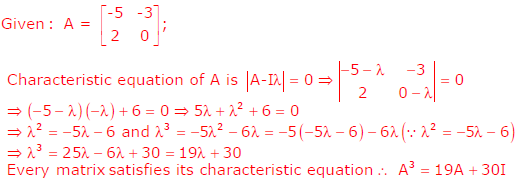# Engineering Mathematics - Online Test

Q1. Roots of the algebraic equation x3 + x2 + x + 1 = 0 are
Explaination / Solution:Q2. With K as a constant, the possible solution for the first order differential equationis
Explaination / Solution:Q3. The function f(x) = 2x - x2 - x3 + 3  has
Explaination / Solution: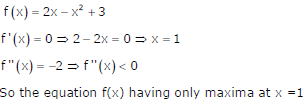Q4. The matrix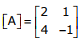is decomposed into a product of a lower triangular matrix [L] and an upper triangular matrix [U]. The properly decomposed [L] and [U] matrices respectively are
Explaination / Solution:

[A] = [L][U] Option D is correct

Q5. The two vectors [1,1,1] and [1, a, a2], where a =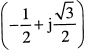, are
Explaination / Solution:

Dot product of two vectors = 1 + a + a= 0
So orthogonal

Q6. Two independent random variables X and Y are uniformly distributed in the interval [–1,1]. The probability that max[X, Y] is less than 1/2 is
Explaination / Solution: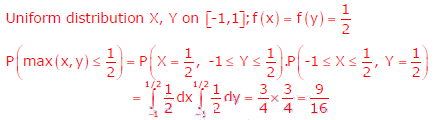Q7. If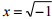, then the value of xx is
Explaination / Solution: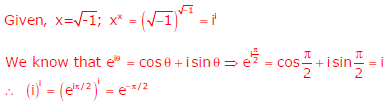Q8. With initial condition x(1) = 0.5, the solution of the differential equation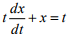is
Explaination / Solution:Q9. The maximum value of F(x) = x3 – 9x2 + 24x + 5 in the interval [1, 6] is
Explaination / Solution: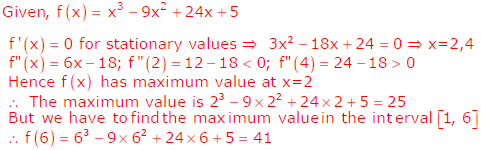Q10.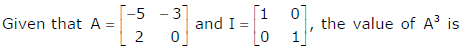Explaination / Solution: